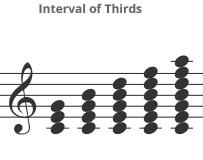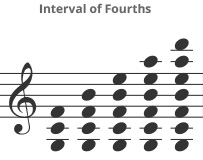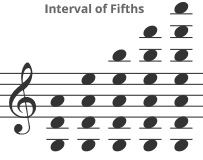« Back to appendix

The triad is the basis for chords, however, the addition of notes at the interval of a third above these triads can create common chords based on the original triad.

A triad, or three notes with the interval of a (major) third over the root (or bottom) note and a second interval of a (minor) third above that. It should be noted that this results in the interval of a perfect fifth above the root (or bottom note).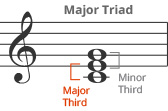seventh chord
A seventh chord, that contains the original triad with an additional note of a (major) third above the basic triad. It is important to understand that that the seventh chord refers to the interval of a seventh between the root (or bottom) note and the additional note above the triad.ninth chord
A ninth chord, with an additional note of a (minor) third above the seventh chord. Again, the ninth chord refers to the interval of a ninth between the root (or bottom) note and the additional note above the seventh chord.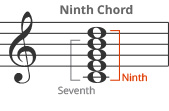eleventh chord
An eleventh chord, with an additional note of a (minor) third above the ninth chord. Again, the eleventh chord refers to the interval of an eleventh between the root (or bottom) note and the additional note above the ninth chord.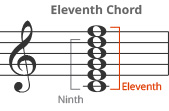thirteenth chord
Thirteenth chord, with an additional note of a (major) third above the eleventh chord. Again, the thirteenth chord refers to the interval of a thirteenth between the root (or bottom) note and the additional note above the eleventh chord.Chords are typically classified as collections of similar intervals that are typically sounded simultaneously. All of the examples above have used the interval of a third (which is the most conmon type of chord in Western music). However, chords can be based not only on the interval of a third, but also on the interval of a fourth, or interval of a fifth.